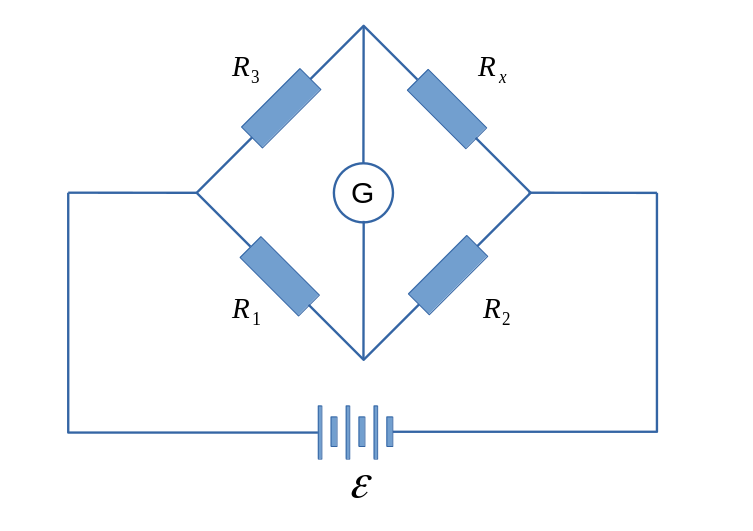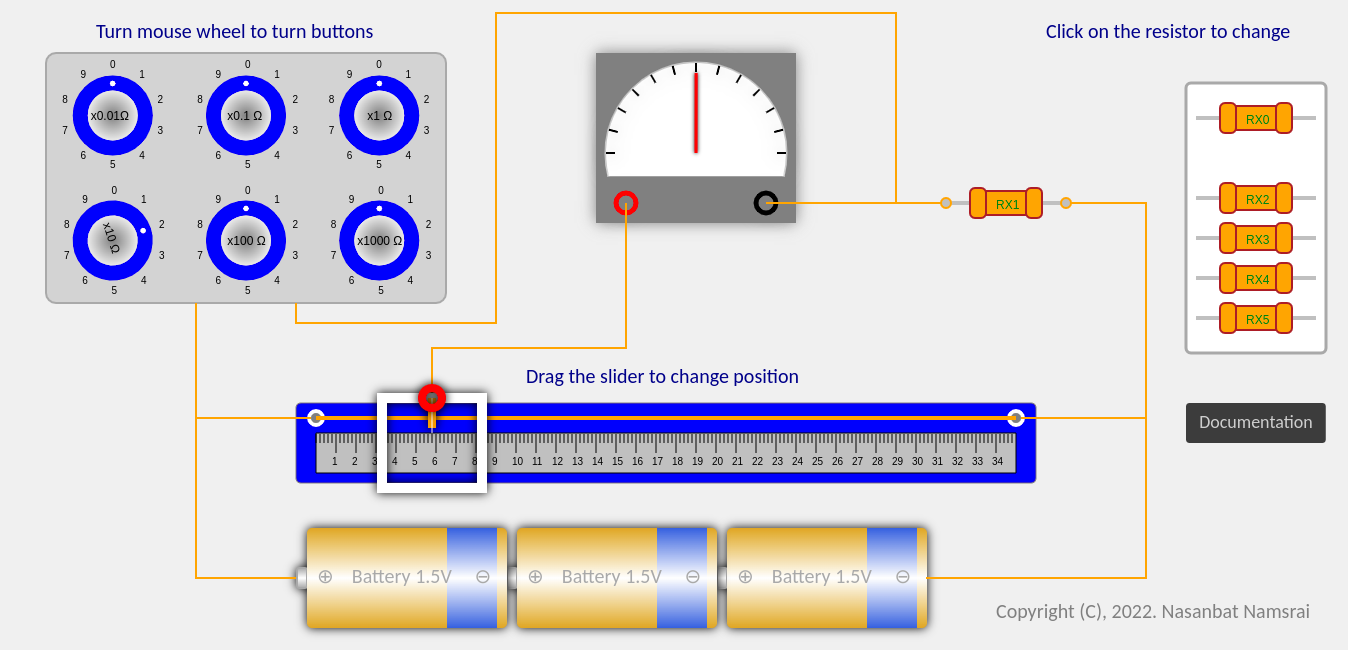# Wheatstone Bridge Experiment Sheet

## Schematic of the Wheatstone Bridge$R_1$ - Resistance of left side of the string.
$R_2$ - Resistance of right side of the string.
$R_3$ - Resistance of the resistor box.
$R_x$ - Unknown resitor.

The implementation of the above schematic is shown here:Resistance of the wire is directly proporional to it's length, $$R_1 = \rho \frac{l_1}{S}, \qquad R_2 = \rho \frac{l_2}{S}$$ Here $S$ is cross sectional area of the string, $\rho$ is resistivity of the string material, $l_1$ and $l_2$ are lengths of right and left side of the strings respectively. Resistance of unknown resistor could be obtained by following formula when bridge is balanced: $$R_x = \frac{R_2}{R_1} R_3 = \frac{l_2}{l_1} R_3$$ Length of the ruler is $l = 35$cm.

Experiment sheet:

 # $R_3$ [$\Omega$] $l_1$ [cm] $l_2 = l - l_1$ [cm] $R_{x}$ [$\Omega$] 1 2 3 4 5 6